International Tables for Crystallography (2006). Vol. D, ch. 3.1, pp. 338-376https://doi.org/10.1107/97809553602060000642

## Contents

• 3.1. Structural phase transitions  (pp. 338-376)
• 3.1.1. Introduction  (pp. 338-340) | html | pdf |
• 3.1.2. Thermodynamics of structural transitions  (pp. 340-349) | html | pdf |
• 3.1.2.1. Introduction  (p. 340) | html | pdf |
• 3.1.2.2. Basic ideas of Landau's theory of phase transitions  (pp. 340-344) | html | pdf |
• 3.1.2.2.1. Description of a prototype example  (p. 340) | html | pdf |
• 3.1.2.2.2. Basic assumptions and strategy  (p. 341) | html | pdf |
• 3.1.2.2.3. Symmetry constraints and form of the free energy  (p. 341) | html | pdf |
• 3.1.2.2.4. Reduction of the number of relevant degrees of freedom: order parameter  (pp. 341-342) | html | pdf |
• 3.1.2.2.5. Stable state below T c and physical anomalies induced by the transition  (pp. 342-343) | html | pdf |
• 3.1.2.2.6. Symmetry considerations  (pp. 343-344) | html | pdf |
• 3.1.2.2.6.1. Order-parameter symmetry  (p. 343) | html | pdf |
• 3.1.2.2.6.2. Degeneracy of the low-symmetry phase  (pp. 343-344) | html | pdf |
• 3.1.2.3. Free-energy models for discontinuous transitions  (pp. 344-345) | html | pdf |
• 3.1.2.4. Generalization of the approach  (pp. 345-347) | html | pdf |
• 3.1.2.4.1. Description of the phase transition  (p. 346) | html | pdf |
• 3.1.2.4.2. Order parameter  (pp. 346-347) | html | pdf |
• 3.1.2.4.3. Stable states and symmetry in the vicinity of(p. 347) | html | pdf |
• 3.1.2.5. Application to the structural transformation in a real system  (pp. 347-349) | html | pdf |
• 3.1.2.5.1. Nature of the groups and of their irreducible representations  (p. 347) | html | pdf |
• 3.1.2.5.2. The example of gadolinium molybdate, Gd 2 (MoO 4 ) 3   (pp. 347-349) | html | pdf |
• 3.1.2.5.2.1. Experimental identification of the order-parameter symmetry  (pp. 347-348) | html | pdf |
• 3.1.2.5.2.2. Construction of the free energy and stable states  (pp. 348-349) | html | pdf |
• 3.1.2.5.2.3. Macroscopic behaviour of GMO  (p. 349) | html | pdf |
• 3.1.3. Equitranslational phase transitions. Property tensors at ferroic phase transitions  (pp. 350-361) | html | pdf |
• 3.1.3.1. Equitranslational phase transitions and their order parameters  (p. 350) | html | pdf |
• 3.1.3.2. Property tensors at ferroic phase transitions. Tensor parameters  (pp. 350-355) | html | pdf |
• 3.1.3.3. Tables of equitranslational phase transitions associated with irreducible representations  (pp. 355-360) | html | pdf |
• 3.1.3.3.1. Explanation of Table 3.1.3.1  (pp. 358-360) | html | pdf |
• 3.1.3.4. Examples  (pp. 360-361) | html | pdf |
• 3.1.4. Example of a table for non-equitranslational phase transitions  (p. 361) | html | pdf |
• 3.1.5. Microscopic aspects of structural phase transitions and soft modes  (pp. 361-372) | html | pdf |
• 3.1.5.1. Introduction  (p. 361) | html | pdf |
• 3.1.5.2. Displacive phase transitions  (pp. 361-372) | html | pdf |
• 3.1.5.2.1. Landau–Devonshire theory  (pp. 361-362) | html | pdf |
• 3.1.5.2.2. Soft modes  (pp. 362-363) | html | pdf |
• 3.1.5.2.3. Strontium titanate, SrTiO 3   (pp. 363-365) | html | pdf |
• 3.1.5.2.4. Lanthanum aluminate, LaAlO 3   (p. 365) | html | pdf |
• 3.1.5.2.5. Potassium nitrate, KNO 3   (p. 365) | html | pdf |
• 3.1.5.2.6. Lanthanum pentaphosphate  (pp. 365-366) | html | pdf |
• 3.1.5.2.7. Barium manganese tetrafluoride  (pp. 366-367) | html | pdf |
• 3.1.5.2.8. Barium sodium niobate  (p. 367) | html | pdf |
• 3.1.5.2.9. Tris-sarcosine calcium chloride (TSCC)  (pp. 367-368) | html | pdf |
• 3.1.5.2.10. Potassium dihydrogen phosphate, KH 2 PO 4   (pp. 368-369) | html | pdf |
• 3.1.5.2.11. Sodium nitrite, NaNO 2   (pp. 369-370) | html | pdf |
• 3.1.5.2.12. Fast ion conductors  (p. 370) | html | pdf |
• 3.1.5.2.13. High-temperature superconductors  (pp. 370-372) | html | pdf |
• 3.1.5.3. Low-temperature ferroelectric transitions  (p. 372) | html | pdf |
• 3.1.6. Group informatics and tensor calculus  (pp. 372-374) | html | pdf |
• 3.1.7. Glossary  (p. 374) | html | pdf |
• References | html | pdf |
• Figures
• Fig. 3.1.2.1. Model of a structural transition  (p. 340) | html | pdf |
• Fig. 3.1.2.2. ( a ) Variation of the free energy as function of the amplitude of the displacement of the central ion in Fig. 3.1.2.1  (p. 340) | html | pdf |
• Fig. 3.1.2.3. Plots representative of the equations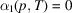and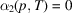(p. 342) | html | pdf |
• Fig. 3.1.2.4. Plots of the Landau free energy as a function of the order parameter, for values of the temperature above or belowor coincident with(p. 342) | html | pdf |
• Fig. 3.1.2.5. ( a ) Qualitative temperature dependence of the specific heat at a continuous transition  (p. 343) | html | pdf |
• Fig. 3.1.2.6. ( a ) Plots of the free energy as a function of the order parameter for various temperature values in the framework of the model of a discontinuous transition associated with equation (3.1.2.13)  (p. 345) | html | pdf |
• Fig. 3.1.2.7. Plots of the free energy as a function of the order parameter for various temperatures in the framework of the model of a discontinuous transition associated with equation (3.1.2.16)  (p. 345) | html | pdf |
• Fig. 3.1.2.8. Schematic representation of the displacement associated with the order parameter in a crystal having trigonal (rhombohedral) symmetry  (p. 345) | html | pdf |
• Fig. 3.1.2.9. Rotations/reflections belonging to the point group of gadolinium molybdate  (p. 348) | html | pdf |
• Fig. 3.1.2.10. Temperature dependence of the macroscopic susceptibility (or elastic compliance,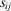) in gadolinium molybdate  (p. 349) | html | pdf |
• Fig. 3.1.3.1. Lattice of subgroups of the group(p. 359) | html | pdf |
• Fig. 3.1.3.2. Lattice of subgroups of the group(p. 359) | html | pdf |
• Fig. 3.1.5.1. Free energy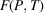and order parameter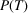from the Landau–Devonshire theory [equation (3.1.5.1 a )] for a continuous second-order ferroelectric phase transition  (p. 363) | html | pdf |
• Fig. 3.1.5.2. Free energyand order parameterfrom the Landau–Devonshire theory [equation (3.1.5.1 a )] for a discontinuous first-order ferroelectric phase transition  (p. 363) | html | pdf |
• Fig. 3.1.5.3. Three-dimensional graph of phase boundaries as functions of temperature T , pressure p and applied electric field E , showing a tricritical point where three continuous phase boundaries intersect  (p. 363) | html | pdf |
• Fig. 3.1.5.4. Structure of strontium titanate above (undisplaced ions) and below (arrows) its anti-ferrodistortive phase transition at ca. 105 K  (p. 364) | html | pdf |
• Fig. 3.1.5.5. Rotation angle versus temperature for the oxygen octahedron distortion below 105 K in strontium titanate described in Fig. 3.1.5.4  (p. 364) | html | pdf |
• Fig. 3.1.5.6. Raman spectra of strontium titanate below its cubic–tetragonal phase transition temperature  (p. 364) | html | pdf |
• Fig. 3.1.5.7. Temperature dependence of phonon branches observed in the Raman spectra of tetragonal strontium titanate  (p. 364) | html | pdf |
• Fig. 3.1.5.8. ( a ) Structure of lanthanum aluminate above (undistorted) and below (arrows) its cubic–rhombohedral phase transition near 840 K  (p. 365) | html | pdf |
• Fig. 3.1.5.9. Phase diagram of potassium nitrate, KNO 3   (p. 365) | html | pdf |
• Fig. 3.1.5.10. ( a ) Soft' optical phonon frequency versus temperature in LaP 5 O 14 , showing displacive character of the phase transition  (p. 366) | html | pdf |
• Fig. 3.1.5.11. ( a ) Structure of barium metal fluoride Ba M F 4 ( M = Co, Mn, Mg, Zn, Ni) at ambient temperature (300 K)  (p. 366) | html | pdf |
• Fig. 3.1.5.12. Structure of the tungsten bronze barium sodium niobate Ba 2 NaNb 5 O 15 in its highest-temperaturephase above 853 K  (p. 367) | html | pdf |
• Fig. 3.1.5.13. Sequence of phases encountered with raising or lowering the temperature in barium sodium niobate  (p. 367) | html | pdf |
• Fig. 3.1.5.14. Structure of tris-sarcosine calcium chloride, (CH 3 NHCH 2 COOH) 3 CaCl 2   (p. 368) | html | pdf |
• Fig. 3.1.5.15. Soft' optical phonon frequencies versus temperature in both ferroelectric and paraelectric phases of tris-sarcosine calcium chloride  (p. 368) | html | pdf |
• Fig. 3.1.5.16. The structure of potassium dihydrogen phosphate, KH 2 PO 4 , showing the OHO hydrogen bonds  (p. 368) | html | pdf |
• Fig. 3.1.5.17. Double-well models  (p. 369) | html | pdf |
• Fig. 3.1.5.18. Pressure dependence of the soft' optical phonon branch Raman spectra in potassium dihydrogen phosphate  (p. 369) | html | pdf |
• Fig. 3.1.5.19. Structure of sodium nitrite, NaNO 2   (p. 369) | html | pdf |
• Fig. 3.1.5.20. Raman spectra of sodium nitrite, showing diffusive Debye-like response due to large-amplitude flopping over of nitrite ions  (p. 370) | html | pdf |
• Fig. 3.1.5.21. Phase diagram for sodium nitrite for conjugate' electric fields applied along the polar b axis, showing triple point, tricritical point and critical end point  (p. 370) | html | pdf |
• Fig. 3.1.5.22. Phase diagram for sodium nitrite for electric fields applied perpendicular to the polar b axis  (p. 370) | html | pdf |
• Fig. 3.1.5.23. ( a ) Crystal structure of silver iodide tungstate (Ag 13 I 9 W 2 O 8 ); ( b ) showing conduction paths for Ag ions  (p. 371) | html | pdf |
• Fig. 3.1.5.24. Evidence for three phase transitions in silver iodide tungstate: ( a ) dielectric and conductivity data; ( b ) specific heat data; ( c ) Raman data  (p. 371) | html | pdf |
• Fig. 3.1.5.25. Raman spectra of YBa 2 Cu 3 O 7− x below an apparent phase transition at ca. 235 K  (p. 372) | html | pdf |
• Tables
• Table 3.1.1.1. Ferroic classification of structural parameters  (p. 339) | html | pdf |
• Table 3.1.2.1. Transformation of the components ofunder the symmetry operations of group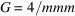(p. 343) | html | pdf |
• Table 3.1.2.2. Matrices defining the irreducible representations offor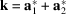(p. 348) | html | pdf |
• Table 3.1.2.3. Action of the generators ofon the order parameter and on the polarization and strain components  (p. 349) | html | pdf |
• Table 3.1.3.1. Point-group symmetry descents associated with irreducible representations  (pp. 352-357) | html | pdf |
• Table 3.1.3.2. Symmetry descents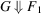associated with two irreducible representations  (p. 358) | html | pdf |
• Table 3.1.3.3. Important property tensors  (p. 360) | html | pdf |
• Table 3.1.4.1. Possible symmetry changes across transitions from a parent phase with space group,,,,or(p. 362) | html | pdf |
• Table 3.1.5.1. Low-temperature ferroelectrics  (p. 372) | html | pdf |